## Explain Conduction in Intrinsic Semiconductors:

Explain Conduction in Intrinsic Semiconductors – As already explained, conduction through a semiconductor is due to two mechanisms viz. drift and diffusion. The movement of charge carriers (electrons and holes) under the influence of an applied electric field E is termed as the drift current.

The conductivity of σe of the semiconductor due to electrons in conduction band is given as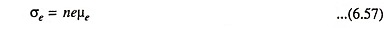where n is the number of electrons per unit volume of the conductor (i.e., electron density or concentration), e is the electron charge and μe is the electron mobility.

Similarly, the conductivity σh of the semiconductor due to holes is given as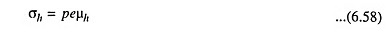where p is the number of holes per unit volume and μh is the hole mobility.

Since Conduction in Intrinsic Semiconductors is by free electrons as well as by holes, total conductivity σ is given as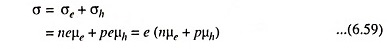Since in intrinsic semiconductors n = p = ni, the Conduction in Intrinsic Semiconductors of holes or free electrons in the semiconductor, intrinsic semiconductor conductivity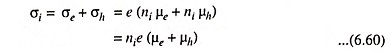Current density,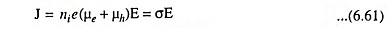Current,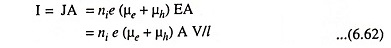where E is applied electric field, V is the applied potential difference across the two ends of the conductor and A is the cross-sectional area of the conductor.

In case of N-type semiconductor hole concentration ph is negligible and electron concentration nn = ND. So conductivity in N-type semiconductor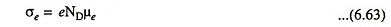Similarly in case of P-type semiconductor electron concentration is negligible and hole concentration ph = NA. So conductivity in P-type semiconductor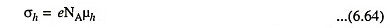Total current density due to drift of electrons and holes on application of electric field E,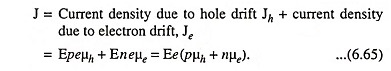Intrinsic Concentration: It has been established that the intrinsic concentration ni varies with temperature according to the relation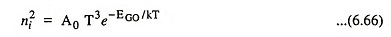where A0 is a constant and is independent of temperature, k is a Boltzmann constant in eV per K and EGO is the energy gap in electron volts at zero absolute temperature, T is temperature in degrees absolute and ni is concentration in number per cubic metre. A0 is taken as 2.33 x 1043.

Mobility: The mobility μ of charge carriers (electrons and holes) varies as T-m over a temperature range of 100 K and 400 K. For silicon m = 2.5 for electrons and 2.7 for holes. For germanium m = 1.66 for electrons and 2.33 for holes.

The carrier currents are also due to concentration gradients in the doped material which leads to diffusion of carriers from high concentration region to low concentration region.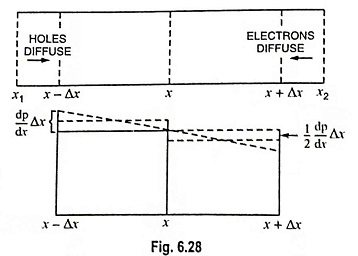A concentration gradient exists if either the number of holes or electrons is greater in one region as compared to the rest of the region. As already explained, when a concentration gradient of carriers exists in a material, the charge carriers tend to move from the region of high concentration to the region of lower concentration. The process is called the diffusion and the current due to this process is called the diffusion current.

Let us assume that at reference plane x, the density of holes is p per m3. Let the carriers have a mean free path of Δx and mean free time of Δt between collisions. Assuming uniform density gradient of holes as -dp/dx.

The average density of holes in the region x – Δx and x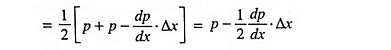Flow of holes to the right per unit time,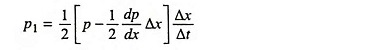Left directed hole flow across reference plane x per unit time,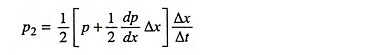The net rate of flow of holes is to the right and is given as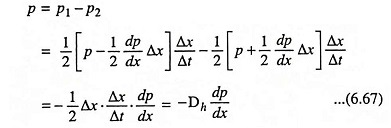where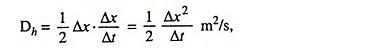called the diffusion current.

The current density due to diffusion of holes is given as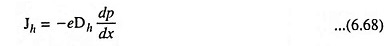Similarly current density due to diffusion of electrons is given as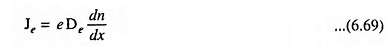Total Current in a Semiconductor: It is possible that a potential gradient and a concentration gradient may exist simultaneously within a semiconductor. In such a case, the total current is the sum of drift current due to potential gradient and the diffusion current due to charge carrier concentration gradient.

Thus,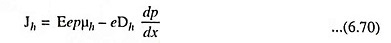and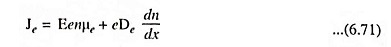The constants EG0, μe, μh, De, Dh and many other important physical quantities for silicon and germanium are given in tabular form (Table 6.4).

Einstein Relationship: Diffusion constant D and mobility μ are statistical thermodynamic phenomena. Hence they are not independent of each other. The diffusion constants Dh and De and mobilities μh and μe are related by Einstein’s equation given aswhere VT is the volt-equivalent of temperature and is defined as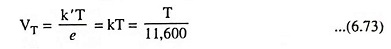where k′ is Boltzmann constant in joule/K and k is Boltzmann constant in eV/ K.

At room temperature (300 K)### Properties of Intrinsic Semiconductors:

The important properties of silicon and germanium are given below in tabular form (Table 6.4)

Scroll to Top